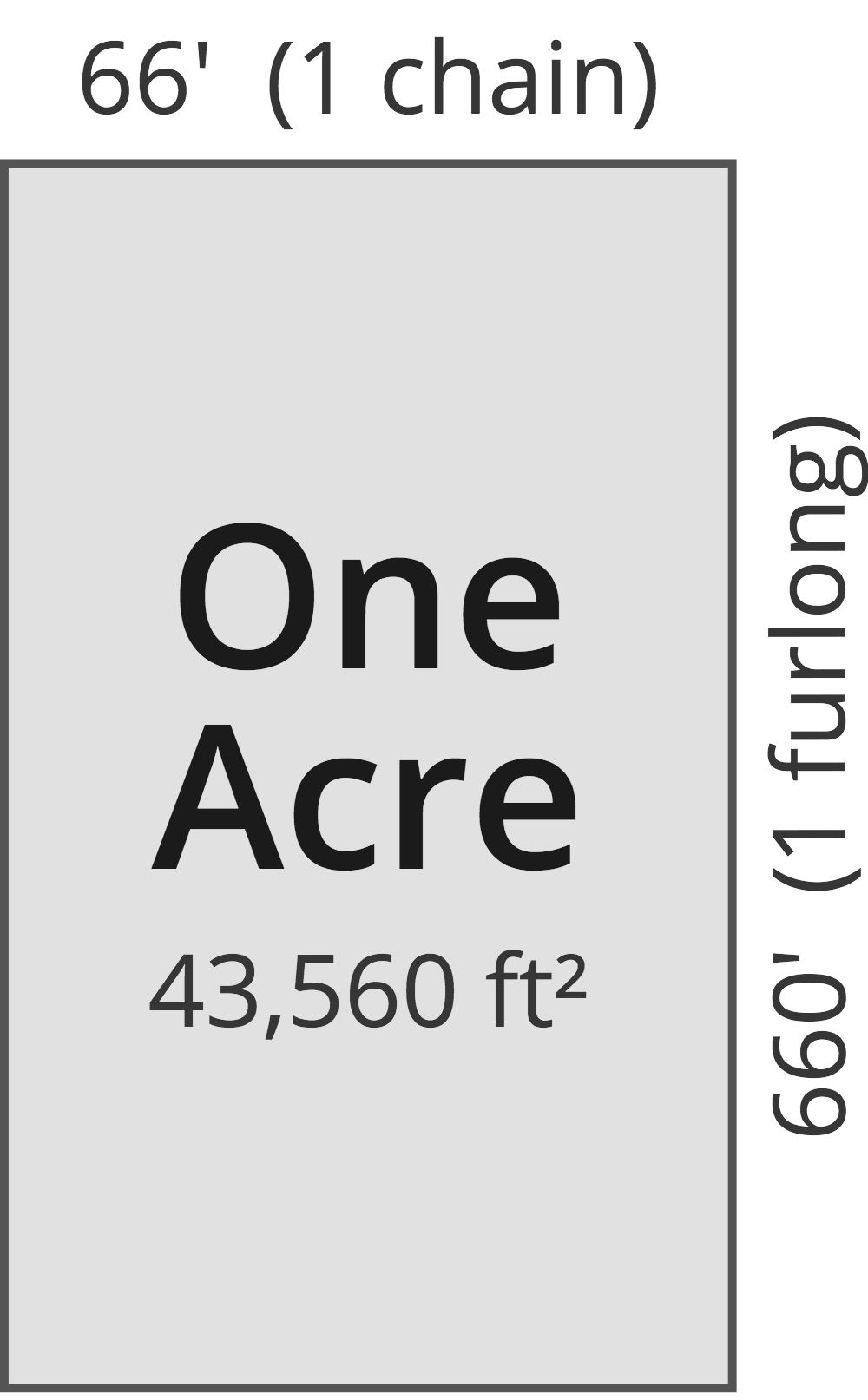# Square Inches to Acres Converter

Enter the area in square inches below to get the value converted to acres.

Results in Acres:1 sq in = 1.5942E-7 ac
Hint: use a scientific notation calculator to convert E notation to decimal

Do you want to convert acres to square inches?

## How to Convert Square Inches to Acres

To convert a measurement in square inches to a measurement in acres, divide the area by the following conversion ratio: 6,272,640 square inches/acre.

Since one acre is equal to 6,272,640 square inches, you can use this simple formula to convert:

acres = square inches ÷ 6,272,640

The area in acres is equal to the area in square inches divided by 6,272,640.

For example, here's how to convert 5,000,000 square inches to acres using the formula above.
acres = (5,000,000 sq in ÷ 6,272,640) = 0.797113 ac

## What Is a Square Inch?

One square inch is equivalent to the area of a square with sides that are each 1 inch in length. One square inch is equal to 1/144 of a square foot or 1/1,296 of a square yard.

The square inch is a US customary and imperial unit of area. A square inch is sometimes also referred to as a square in. Square inches can be abbreviated as sq in, and are also sometimes abbreviated as in². For example, 1 square inch can be written as 1 sq in or 1 in².

## What Is an Acre?

One acre is defined as the area equal to a space that is one chain (66 ft) by one furlong (660 ft), or 10 square chains. That's equal to 43,560 square feet or 1/640 of a square mile for those unfamiliar with those units of measure.The acre is a US customary and imperial unit of area. Acres can be abbreviated as ac; for example, 1 acre can be written as 1 ac.

You can use an acreage calculator to measure the area of a plot of land in acres by locating the boundaries on a map.

## Square Inch to Acre Conversion Table

Table showing various square inch measurements converted to acres.
Square Inches Acres
1 sq in 0.00000015942 ac
2 sq in 0.00000031885 ac
3 sq in 0.00000047827 ac
4 sq in 0.00000063769 ac
5 sq in 0.00000079711 ac
6 sq in 0.00000095654 ac
7 sq in 0.000001116 ac
8 sq in 0.0000012754 ac
9 sq in 0.0000014348 ac
10 sq in 0.0000015942 ac
100 sq in 0.000015942 ac
1,000 sq in 0.000159 ac
10,000 sq in 0.001594 ac
100,000 sq in 0.015942 ac
1,000,000 sq in 0.159423 ac
10,000,000 sq in 1.5942 ac

## References

1. National Institute of Standards and Technology, Specifications, Tolerances, and Other Technical Requirements for Weighing and Measuring Devices, Handbook 44 - 2019 Edition, https://nvlpubs.nist.gov/nistpubs/hb/2019/NIST.HB.44-2019.pdf